Inheritance

# Virtual Inheritance §

This is pretty cool. Introduced in CS247. I didn’t know this was a thing.

Virtual inheritance is a C++ technique that ensures only one copy of a base class’s member variables are inherited by grandchild derived classes.

https://stackoverflow.com/questions/3414639/where-is-the-virtual-keyword-necessary-in-a-complex-multiple-inheritance-hiera

Virtual Inheritance helps us fix the Diamond Problem.

Syntax

// struct B: A becomes:
struct B: virtual A {...}

// class C: public A becomes:
class C: virtual public A {...}

The keyword virtual indicates that this base class will be shared among all others who inherit from it virtually in the inheritance hierarchy.

Now, dOjb.a unambiguous, a field is now shared between both patterns of the object.

class A {
public:
A(int x) {...}
}
class B: public virtual A {
public:
B(...): A{1} ... {}
};

class C: public virtual A {
public:
C(...): A {2} {...}
};

class D: public B, public C {...}

Constructors for virtual bases must run before any other constructors.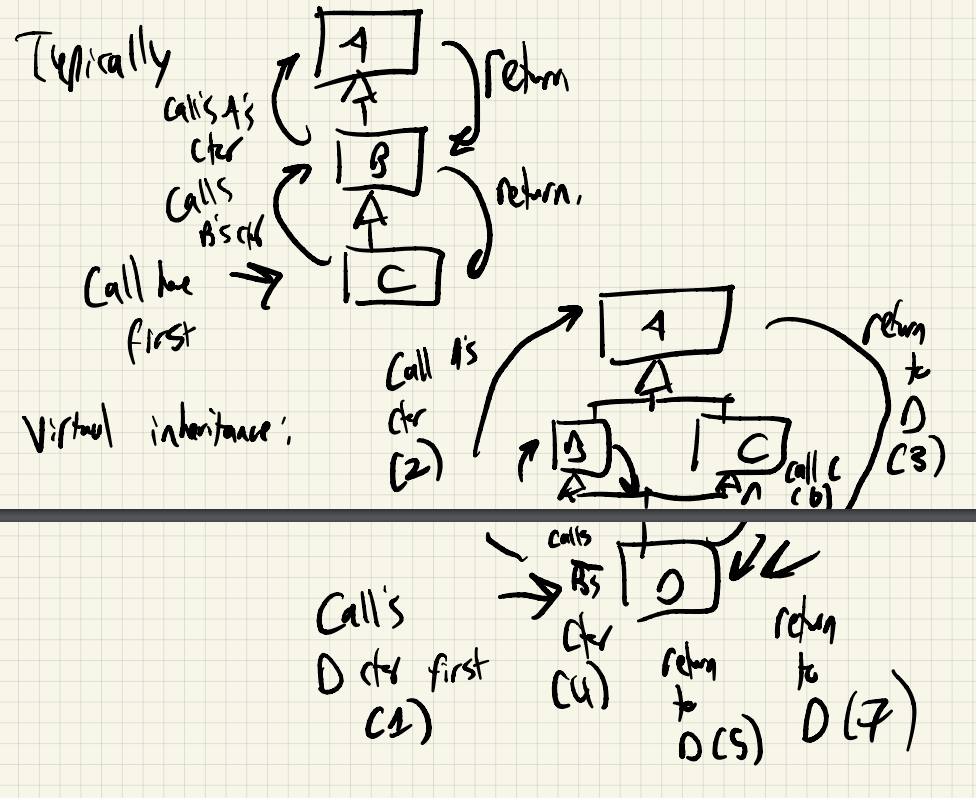• This shows the order in which the objects is constructed for virtual inheritance:
1. Calls D’s constructor
2. Calls A’s constructor
3. Returns to D
4. Calls B’s constructor
5. Returns to D
6. Calls C’s constructor
7. returns to D

Fixed

class A {
public:
A(int x): ... {...}
};

class B: public virtual A {
public:
B(): ... {...} // Calls A's ctor
};

class C: public virtual A {
public:
C(): ... {...} // Calls A's ctor
};

class D: public B, public C {
public:
D(): A{5}, B{}, C{} ... {...}
};

#### Object Layout with Virtual Inheritance §

How does object layout work with multiple virtual inheritance?

class A {...}
class B: public A{...}

B object: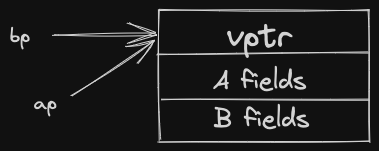B* bp = new B{...};
A* ap = bp;

To treat a B* like an A*, simply create a pointer copy, points at the same location, ignore B fields. Same strategy cannot be used for multiple virtual inheritance.

D* dp = new D{...};
A* ap = dp;
B* bp = dp;
C* cp = dp; // Doesn't look like a Cobject if we point at the same location!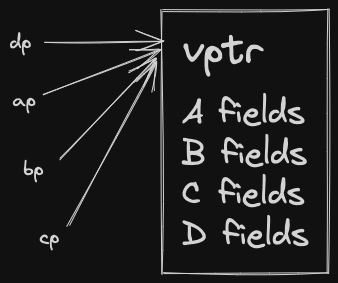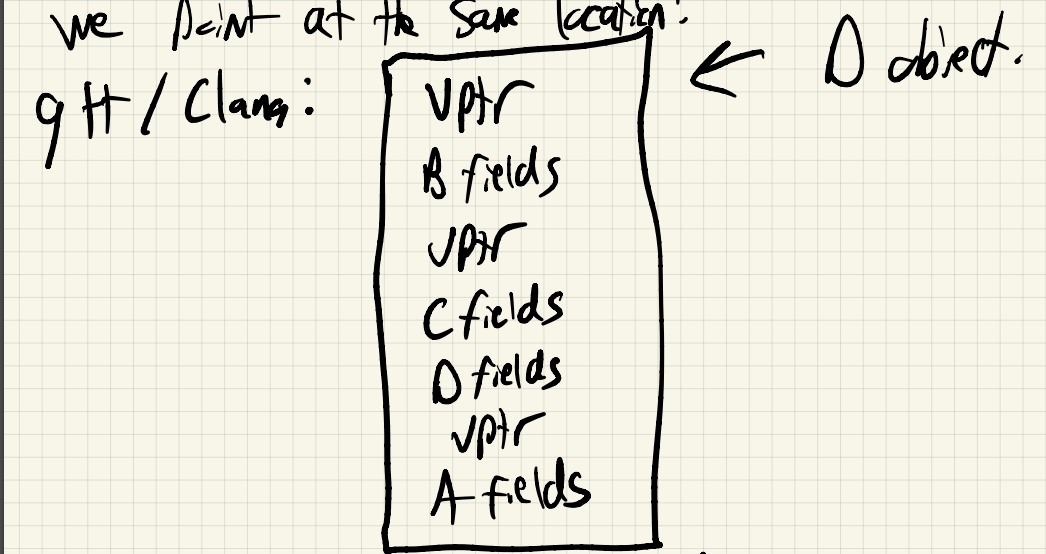Note

virtual Base(A) is actually stored at the end of the object! Not the beginning.

B* bp = ...;
cout << bp->a << endl;

If bp points at a D object, see above memory diagram. If bp points at a B object,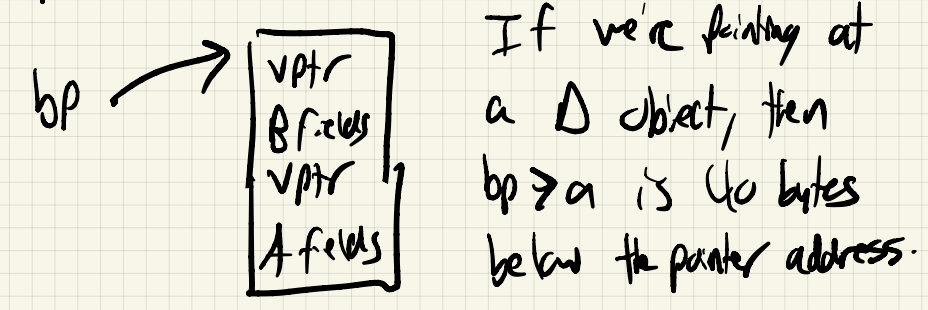Note

• If we’re pointing at a D object, bp->a is 40 bytes below the pointer address.
• If we’re pointing at a B object, bp->a is 24 bytes below the pointer address.

Note: Now, finding superclass fields depends on the dynamic type.

Solution: Store the offset to the superclass fields inside of the vtable.

This is where the new “virtual” inheritance comes from.

Note: The D object does not look like an A object, a C object, or a D object simultaneously, but portions of it do.

Doing pointer assignment can change where the pointer is pointing to.

• This is really IMPORTANT, be careful
D* dp = new D{...};
A* ap = dp; // dp points at the top of the D object
// ap points just at the t position
• static_cast / dynamic_cast will also adjust pointer locations for you as necessary.
• reinterpret_cast won’t - underscores danger associated with the cast.

Finally, both Google C++ Style guide, and ISOCpp both recommend when using multiple inheritance, Interface Classes are best.

### Exercise §

#include <iostream>
using namespace std;

struct A {
int a;
A(int a): a{a} { cout << "A ctor called" << endl;}
};

struct B: virtual A {
int b;
B(int b): A{200}, b{b} {cout << "B ctor called" << endl;}
};

struct C: virtual A {
int c;
C(int c): A{300}, c{c} {cout << "C ctor called" << endl;}
};

struct D: B, C {
int d;
D(int d): A{400}, B{500}, C{600}, d{d} { cout << "D ctor called" << endl;}
};

int main() {
D d{247};
cout << d.a << " " << d.b << " " << d.c << " " << d.d << endl;
B b{247};
cout << b.a << " " << b.b << endl;
}

/* OUTPUTo
A ctor called
B ctor called
C ctor called
D ctor called
400 500 600 247
A ctor called
B ctor called
200 247
*/